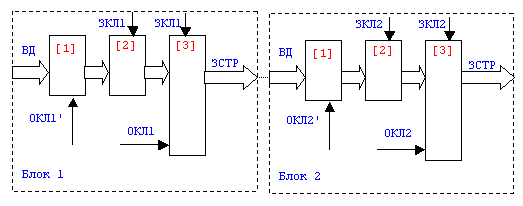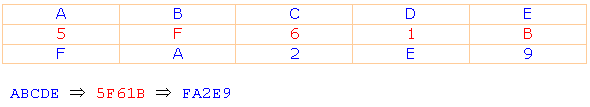Research website of Vyacheslav Gorchilin
Description Ukrainian (Russian) algorithm for secret data transfer

1. The General approach
The algorithm is based on the method of conversion of input data (VD) public-key (OKL), and the method of permutation resulting from the conversion data by the private key (SCL). OKL is transmitted together with VD obtained from the encoded string (SSTR), and its location in TSTR is calculated according to the algorithm with SQL. The last known only to the transmitting and receiving side. Getting VD from str in the reverse order.

The algorithm may consist of one or several blocks, each of which has its own public and private keys. The inclusion of several series-connected units increases the cryptographic strength. In Fig.1 shows two blocks, but next will be described the algorithm of one of them. Others work just as well.

The algorithm is simply implemented on any platform. The platform should support decimal and hexadecimal numbers (summation, subtraction and conversion from one format to another), and have the opportunity to work with substring. Platform limitation is determined only by the size of the VD.

2. Description of the data encryption algorithm
Over 2.1 VD is the algebraic transformation algorithm . This may be a simple summation with OKL', and disable' in the General case can be function from FCL, SCL and other data. To do complex transformations in this part of the algorithm does not make sense, and the task is to mask VD. The data obtained will be called a Line.

2.2 Next, the String is converted by the method of permutation to form a so-called "holes". The holes represent the location in obtained after permutations of the String that have a sequence number, but do not yet have values. The order rearrangement and the formation of holes defines SCL .

2.3 In holes obtained in P2.2, is inserted approx algorithm . Space the holes determines the SCL. Thus SSTR contains the transformed VD and approx.Fig.1

3. Description of the algorithm decrypt data
In Fig.2 shows a diagram of the decryption of the data. The scheme consists of two cascaded blocks, but next will be described the algorithm of one of them. Others work just as well.

3.1 Of SSTR using SQL removed is unknown until approx algorithm .

3.2 SSTR is converted by the method of permutation algorithm . The obtained data then will be called a Line.

3.3 Above the Line is the algebraic transformation algorithm . The result is VD. VD input (Fig.1 Block1) and VD at the output (Fig.2 Option1) are equivalent.Fig.2

4. Generating SQL
SCL cannot be any combination of characters or numbers, it has to be generated by a special algorithm . Moreover, for each block (two-unit example in Fig.1) it needs to be unique. The generation is done once, after which SQL is issued to the transmitting and receiving side, and should be known only to them.

 the Algebraic transformation implies that any algebraic operations on the data:
ST=f(VD,OKL')
where: ST — the resulting algebraic transformations String;
f(VD,OKL') — algebraic function from VD and approx', while in the General case: OKL'=f(FCL,SCL,...);Fig.3
An example of algebraic transformation shown in Fig.3, where VD is equal to ABCDE, and approx' equal 5F61B. The algorithm is based on the addition of two hexadecimal numbers after which it remains one the youngest category, for example: A+5=F C+6=2. As a result of transformation get the String FA2E9, the length of which is equal to the length of VD.

The algorithm for the next permutation. Is a sequence of atomic bust and fitted bodice. Furthermore, k=i until such time as k is less than the length of the SCL. Then k=(i)mod(L), where L is the length of SCL, i.e. quite simply the expense of k starting from scratch each time k reaches L. Swap the i-th element INTEGRATED in the j-th element PSTR according to the rule: j=i+Zk, where Zk is the value k of the element SCL. After moving all INTERNAL we get MID length greater than the INTERNAL length SCL negative one. This means that PSTD has "holes" — i.e. places with no values.Fig.4
An example of getting PSTD from BUILT-in is shown in Fig.4, where SCL equal to 2011 and equal to BR ABCDEFGH. In the result of permutations obtained PSTD equal-BACDFEGH-- , where the dashes '-' marked hole. In the General case, the number of holes is defined as: RR=N-1, where: BH is the number of holes in the line, N is the radix. For example, for SQL consists of four chetyrehrjadnyh, the number of holes, in General, is equal to three.

 the algorithm of the lookup disable in holes like this. In the first left-to-right hole is inserted the first element of OCP, the second second and so on until, until all holes are filled. The number of holes are searched by the algorithm . In the example from  (Fig.4) it will look like: -BACDFEGH-- => 5BACDFEGHD0, where OKL is equal to 5D0.

 algorithm for the extraction of OCPs from SSTR like this. Find the number of holes in the algorithm , hereinafter referred to as ND. Fetching the value from the first LP, and substitute it in place of the first element OKL. In place of the second element OKL substitute the value of the second LP, etc., until you get all the elements OKL. I.e. this algorithm, the backward algorithm .

 This algorithm is permutation — backward algorithm , but in this case INTERNAL — famous Line at the entrance, and MID — yet unknown String at the output of the algorithm. Create a PSTR of zeros (or other values) with a length equal to the length of VSTR minus the number of holes. Produced by a sequential atomic bust and fitted bodice. Thus k=i until such time as k is less than the length of SCL, and then k=(i)mod(L). Substitutable for the i-element VSTR j-th element PSTR according to the rule: i=j-Zk, where Zk is the value k of the element SCL. Thus the output of the algorithm is obtained PSTR length less than the length of VSTR to the number of holes.

VD=f(ST,OKL')
where: VD resulting algebraic inverse transformation data;
f(ST,OKL') — this is an algebraic function of ST and OKL that ST=f(VD,disable'), while in the General case: OKL'=f(FCL,SCL,...); is the same as in .Fig.5
An example of algebraic transformation shown in Fig.5. All actions reverse actions in the example from  (Fig.1). Need of element values of the input to subtract the value of the element OKL (or function from FCL) by the method of additions. Ie if first number is less than another, then the obtained difference is added the number corresponding to the numeral system (for example the hexadecimal number system is 16).

 the Algorithm for generating SQL following. Creates an empty array of 3*N elements and an empty string of N elements, where N is the radix. Generated first random number R0 in the range 0..N-1. Checks for the existence of a non-empty array of element numbers: 0+R0 and N+0+R0. If both elements are empty, the number R0 is considered to be successfully generated, and is stored in a string in place of the number '0' and is stored in the array, numbered 0+R0 and N+0+R0. If at least one of the two check elements of array are non-empty (i.e. it contains the number), the number R of0 is generated and checked again.

In the same way as we generate N numbers. In the General case, the generated number is Ri, and the elements of the array for checking and entering to values: i+Ri and N+i+Riwhere: i — ordinal number of from zero to N-1. The output is a string of N elements, which is equal to SKL.

Vyacheslav Gorchilin, 2004
* Reprinting of the article and the module are possible with the links on the website and respect the copyright